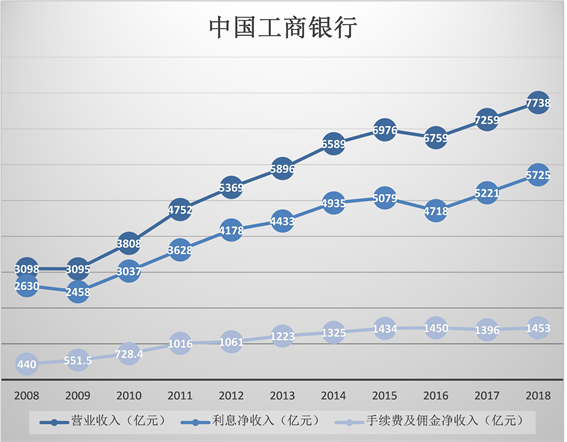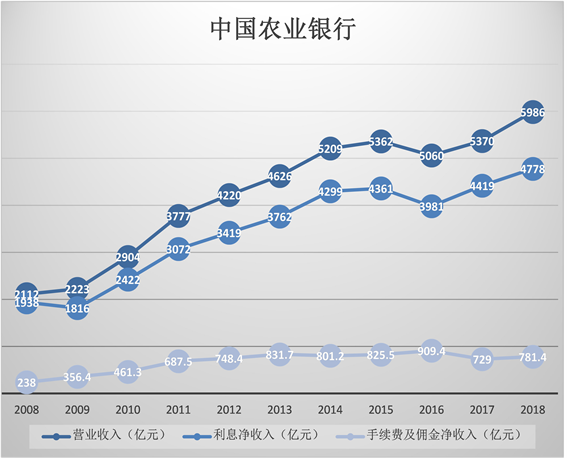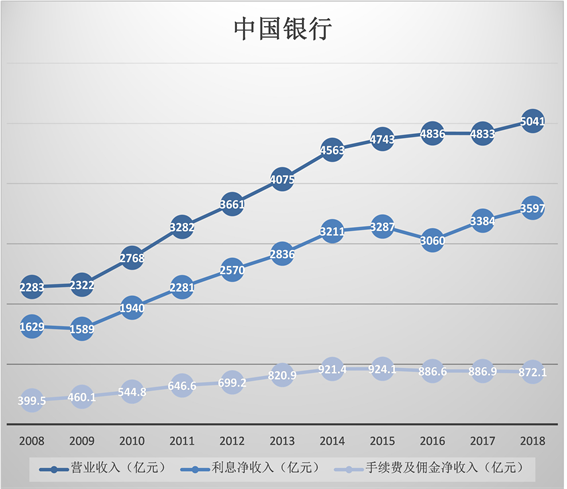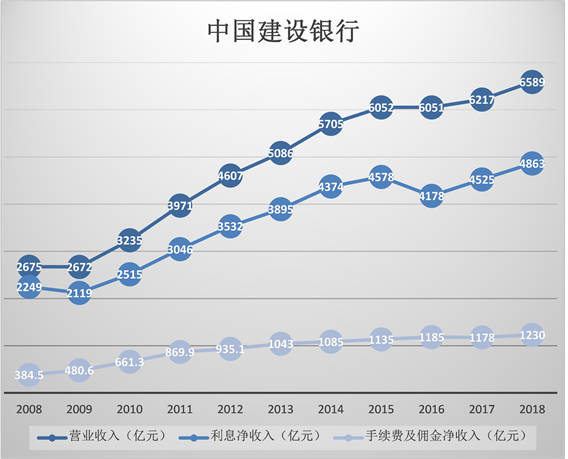# 以做市商模型看利率市场化对商业银行的影响An Application of the Market Maker Model in Analyzing the Influences of Interest Rate Liberalization on Chinese Commercial Banks

DOI: 10.12677/FIN.2020.104041, PDF, HTML, XML, 下载: 168  浏览: 451

Abstract: Without the regulation of interest rate, great changes have taken place in China’s commercial banking sector. Besides, the profit model of China’s commercial bank also develops accordingly. The annual data of China’s commercial bank in the decade from 2008 to 2018 (four banks in China as examples) were selected to analyze the current profit model of China’s commercial bank. And the factors influencing the profit model of China’s commercial bank were theoretically analyzed to demonstrate the function graph of interest rate liberalization on the interest rate and the difference of deposits and loans of Chinese commercial Banks. At the same time, it analyzes the opportunities and challenges brought by the interest rate liberalization for China’s commercial bank and puts forward corresponding proposals.

1. 引言

“如果巴西丛林一只蝴蝶偶然挥动了它的翅膀这可能会在美国得克萨斯州掀起一场龙卷风。”如果我们将我国的商业银行比作是丛林中的这只蝴蝶，它作为特殊的金融企业，具有信用中介、支付中介、信用创造和金融服务功能。宏观政策若是金融体系中的一盘象棋，那么商业银行好比是象棋棋子中的“将”“帅”在政策的传导过程中作为主角起到主导作用。其中利率像是“车”，威力大而受到器重。利率作为货币金融政策传导机制不容小觑的重要环节以及市场资源配置的导向标志，它就像是蝴蝶身上的翅膀，而利率市场化改革，如同蝴蝶挥动翅膀的微动会影响整个宏观经济，市场借由市场主体供求双方决定调整利率再经各个商业银行整合调节来适应基础利率。

2. 文献综述

2.1. 国外文献综述

2.2. 国内文献综述

3. 研究背景与研究方法

3.1. 研究背景

“不以规矩，不成方圆”，二战以后的国际货币体系可谓是一盘散沙，各国货币竞争性贬值，情况危急。本是世界经济领头羊地位的英国在此次战役中受到极大的打击，巨星陨落必有后来者居上，美国依靠贩卖军火储备黄金一步登顶拔得头筹，依靠世界最大债权国的地位使英国利益为本的“凯恩斯计划”石沉大海。金本位制的瓦解成全美元与作为天然货币的硬通货黄金挂钩，在1944年“布雷顿森林协议”颁布后，昔日英镑的辉煌被美元替代；金本位制被新的金汇兑本位制替代；各行其是的汇率制度被以美元黄金挂钩的固定汇率制度替代。混乱的经济现状迎来了复苏的黎明。

3.2. 研究方法

3.2.1. 理论分析

$S={r}_{L}-{r}_{D}=\left({R}_{L}+{m}_{L}-{n}_{L}\right)-\left({R}_{D}+{m}_{D}-{n}_{D}\right)$

$S={r}_{L}-{r}_{D}=\left({R}_{L}+{m}_{L}-{n}_{L}\right)-\left({R}_{D}+{m}_{D}-{n}_{D}\right)$

${N}_{0}={L}_{0}-{D}_{0}$

${A}_{0}={L}_{0}+{M}_{0}$

${V}_{0}={L}_{0}-{D}_{0}+{M}_{0}={N}_{0}+{M}_{0}$

${r}_{N}={r}_{L}\frac{{L}_{0}}{{N}_{0}}-{r}_{D}\frac{{D}_{0}}{{N}_{0}}$

$\because {V}_{0}={L}_{0}-{D}_{0}+{M}_{0}={N}_{0}+{M}_{0}$

$\therefore {r}_{V}={r}_{N}\frac{{N}_{0}}{{V}_{0}}+{r}_{M}\frac{{M}_{0}}{{V}_{0}}$

${V}_{T}=\left(1+{r}_{N}+{Z}_{N}\right){N}_{0}+\left(1+{r}_{M}+{Z}_{M}\right){M}_{0}$

${V}_{T}=\left(1+{r}_{V}\right){V}_{0}+{M}_{0}{Z}_{M}+{N}_{0}{Z}_{N}$

${E}_{\left({V}_{T}\right)}$$\stackrel{¯}{V}$ 领域内进行泰勒级数展开：

$EU\left({V}_{T}\right)=U\left(\stackrel{¯}{V}\right)+\frac{{U}^{″}\left(\stackrel{¯}{V}\right)}{2}\left({N}_{0}^{2}{\sigma }_{N}^{2}+{M}_{0}^{2}{\sigma }_{M}^{2}+2{N}_{0}{M}_{0}{\sigma }_{NM}\right)\triangleq {E}_{\left( V T \right)}$

$\begin{array}{l}{V}_{T}\left(Q=D\right)\\ =\left(1+{r}_{N}+{Z}_{N}\right){N}_{0}+\left(1+{r}_{M}+{Z}_{M}\right){M}_{0}-\left(1+{r}_{D}\right)Q+\left(1+{r}_{M}+{Z}_{M}\right)Q\\ ={V}_{0}\left(1+{r}_{M}\right)+{Z}_{N}{N}_{0}+{Z}_{M}{M}_{0}+\left({r}_{M}-{r}_{D}+{Z}_{M}\right)Q\end{array}$

$\begin{array}{l}{V}_{T}\left(Q=L\right)\\ =\left(1+{r}_{N}+{Z}_{N}\right){N}_{0}+\left(1+{r}_{M}+{Z}_{M}\right){M}_{0}+\left(1+{r}_{l}+{Z}_{L}\right)Q-\left(1+{r}_{M}+{Z}_{M}\right)Q\\ ={V}_{0}\left(1+{r}_{M}\right)+{Z}_{N}{N}_{0}+{Z}_{M}{M}_{0}+\left({r}_{l}-{r}_{M}+{Z}_{l}-{Z}_{M}\right)Q\end{array}$

$\begin{array}{l}EU\left({V}_{T}|Q=D\right)\\ =U\left(\stackrel{¯}{V}\right)+{U}^{\prime }\left(\stackrel{¯}{V}\right)\left({r}_{M}-{r}_{D}\right)Q+\frac{{U}^{″}\left(\stackrel{¯}{V}\right)}{2}\left[\left({r}_{M}-{r}_{D}\right)Q+{\left({N}_{0}-Q\right)}^{2}{\sigma }_{N}^{2}\\ \text{\hspace{0.17em}}\text{\hspace{0.17em}}\text{ }+{\left(Q+{M}_{0}\right)}^{2}{\sigma }_{M}^{2}+2{N}_{0}\left(Q+{M}_{0}\right){\sigma }_{MN}\right]\end{array}$

$\begin{array}{l}EU\left({V}_{T}|Q=L\right)\\ =U\left(\stackrel{¯}{V}\right)+{U}^{\prime }\left(\stackrel{¯}{V}\right)\left({r}_{L}-{r}_{M}\right)Q+\frac{{U}^{″}\left(\stackrel{¯}{V}\right)}{2}\left[\left({r}_{L}-{r}_{M}\right)Q+{\left({N}_{0}+Q\right)}^{2}{\sigma }_{N}^{2}\\ \text{\hspace{0.17em}}\text{\hspace{0.17em}}\text{ }+{\left(Q-{M}_{0}\right)}^{2}{\sigma }_{M}^{2}+2\left(\left({M}_{0}-{N}_{0}-Q\right)Q+{N}_{0}{M}_{0}\right){\sigma }_{MN}\right]\end{array}$

$\lambda \left({n}_{D}\right)={\alpha }_{D}-{\beta }_{D}{n}_{D}$$\lambda \left({n}_{L}\right)={\alpha }_{L}-{\beta }_{L}{n}_{L}$

$\mathrm{max}EU\left(\Delta {V}_{T}\right)=\mathrm{max}\left\{\lambda \left({n}_{D}\right)\left[EU\left({V}_{T}|Q=D\right)-EU\left({V}_{T}\right)\right]+\lambda \left({n}_{L}\right)\left[EU\left({V}_{T}\right)|Q=L\right)-EU\left({V}_{T}\right)\right]\right\}$

3.2.2. 财务报表数据分析

2015年10月24日起金融机构一年期贷款基准利率由4.6%下调至4.35%；一年期存款基准利率1.75%下调至1.5%。2013年7月20日起央行全面放开金融机构贷款利率管制。商业银行贷款利率浮动下限最大临界值为0.9倍基准贷款利率(由4.14%到3.915%)，存款利率浮动上限最大临界值为1.1倍基准存款利率(由1.87%到1.65%)。Figure 1. Financial information of ICBCFigure 2. Financial information of ABCFigure 3. Financial information of BOCFigure 4. Financial information of CCB

4. 结论与建议

4.1. 结论

1) 促进中国商业银行发展中间业务逐渐转型。

2) 促使中国商业银行注重金融产品或服务的层次差异化即不同的产品要制定不同的利率标准，提高核心竞争力扩大商业银行的经营自主权。

3) 促使中国商业银行适应市场变化不断开发新的金融产品减少风险性。

1) 利率存在风险性。利率市场化背景下中国商业银行趋向于拉低存贷款利差，提高存款利率也要注意扩大贷款规模或提高贷款利率以控制资产负债降低风险性。

2) 利率市场化会增加各个银行间的银行竞争压力和经营压力，考研了商业银行的经营能力和盈利能力。

3) 考验了银行的管控风险能力和自主议价定价能力。

4.2. 建议

  Smith, A. (1972) An Inquiry into the Nature and Causes of the Wealth of Nations. The Commercial Press, Beijing, p. 78.  Thomas, H. and Saunders, A. (1981) The Determinants of Banks Interest Margins: Theory and Empirical Evi-dence. Journal of Financial and Quantitative Analysis, 28, 581-600. https://doi.org/10.2307/2330377  Allen, L. (1988) The Determinants of Bank Interest Margins: A Note. The Journal of Financial and Quantitative Analysis, 12, 231-235. https://doi.org/10.2307/2330883  Angbazo, L. (1997) Commercial Bank Net Interest Margins, De-fault Risk, Interest Rate Risk and Off-Balance Sheet Banking. Journal of Banking and Finance, 16, 55-87. https://doi.org/10.1016/S0378-4266(96)00025-8  Maudos, J. and Fernandez, L. (2004) Determinants of Net Interest Margin in the Ethiopian Banking Industry. Journal of Finance and Economics, 5, 53-56.  Maudos, J. and Solis, L. (2009) The Determinants of Net Interest Income in the Mexican Banking System: An Integrated Model. Journal of Banking & Finance, 33, 1920-1931. https://doi.org/10.1016/j.jbankfin.2009.04.012  陆静, 王漪碧, 王捷. 贷款利率市场化对商业银行风险的影响——基于盈利模式与信贷过度增长视角的实证分析[J]. 国际金融研究, 2014(6): 50-56.  胡志九, 楚啸原. 利率市场化对商业银行长期影响刍议[J]. 中央财经大学学报, 2018(6): 87-88.  蒋海, 张小林, 陈创练. 利率市场化进程中商业银行的资本缓冲行为[J]. 中国工业经济, 2018(11): 23-32.  尹雷, 赫国胜. 利率市场化、金融监管与银行危机[J]. 金融论坛, 2013(11): 45-48.